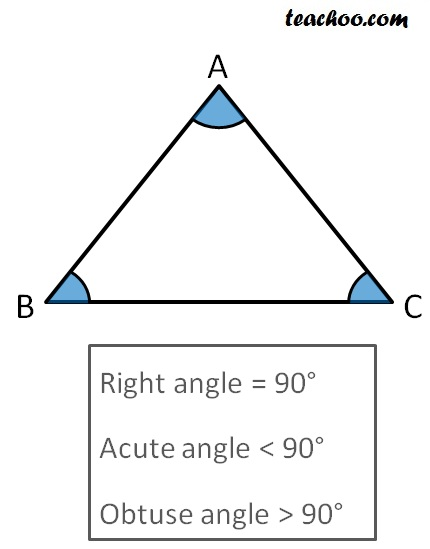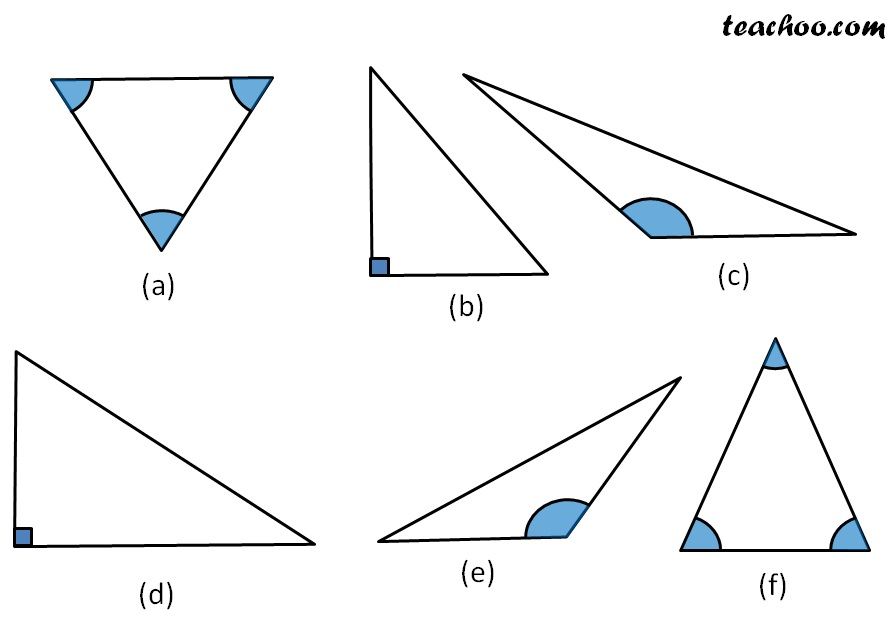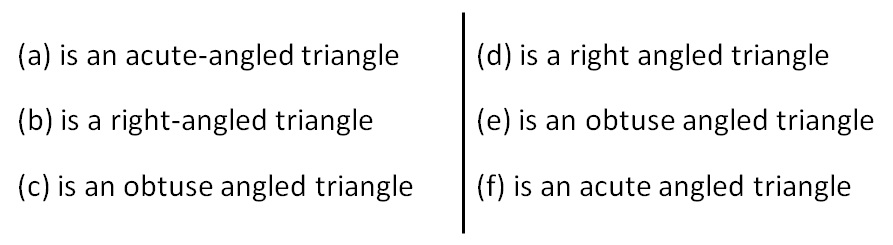##(adsbygoogle = window.adsbygoogle || []).push({});

Now, in ∆ABC,

there can be 3 cases

• All angles are acute
• One angle is right angle (90°)
• One angle is greater than 90° (i.e one angle is obtuse)

If all angles are acute, it is an acute angled triangle.

If one angle is right angle, it is a right angled triangle.

If one angle is greater than 90°, it is an obtuse angled triangle.Subscribe to our Youtube Channel - https://you.tube/teachoo

1. Chapter 6 Class 7 Triangle and its Properties
2. Concept wise
3. Basic Definitions

Transcript

Now, in ∆ABC, there can be 3 cases All angles are acute One angle is right angle (90°) One angle is greater than 90° (i.e one angle is obtuse) If all angles are acute, it is an acute angled triangle. If one angle is right angle, it is a right angled triangle. If one angle is greater than 90°, it is an obtuse angled triangle. (a) is an acute-angled triangle (b) is a right-angled triangle (c) is an obtuse angled triangle (d) is a right angled triangle (e) is an obtuse angled triangle (f) is an acute angled triangle

Basic Definitions